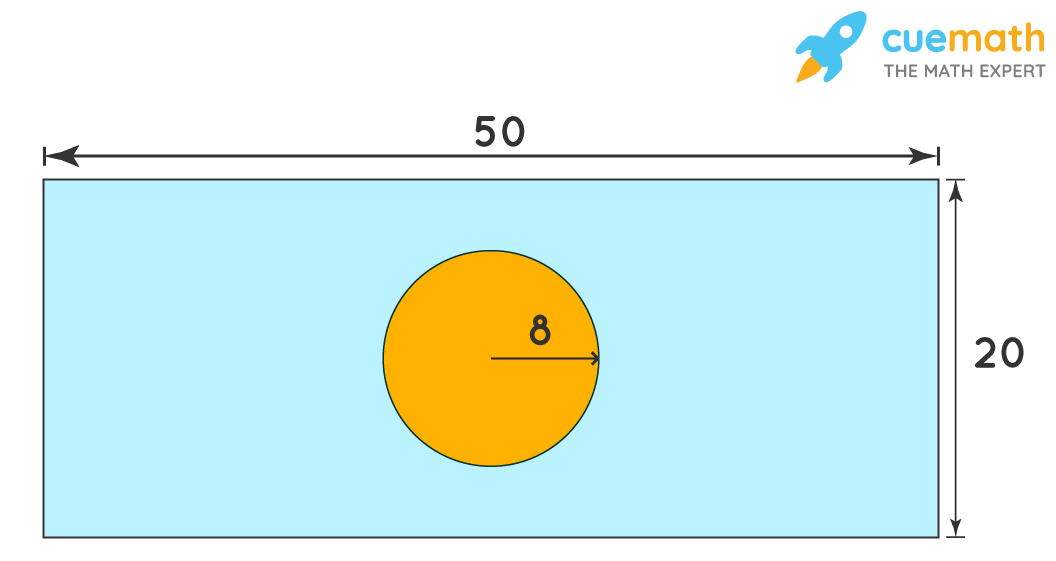# Find the area of the shaded region of a rectangle

## Question: <!--td {border: 1px solid #ccc;}br {mso-data-placement:same-cell;}--> Find the area of the shaded region of a rectangle.The area of the rectangle is equal to the product of its length and breadth and the area of the circle is given by the formula π r2, where r is the radius.

## Answer: (16 π - 100) square units

We will use the formula of the area of a circle to find the area of the circle inside the rectangle and deduct its area from the area of the rectangle.

## Explanation:

Area of Circle = π r2 = π (8)2 = 16 π square units

Area of Rectangle = Length × Breadth = 50 × 20 = 100 square units

Area of Shaded Region = Area of Rectangle - Area of Circle = (16 π - 100) square units